Examples

Chapter 1 Class 11 Sets
Serial order wise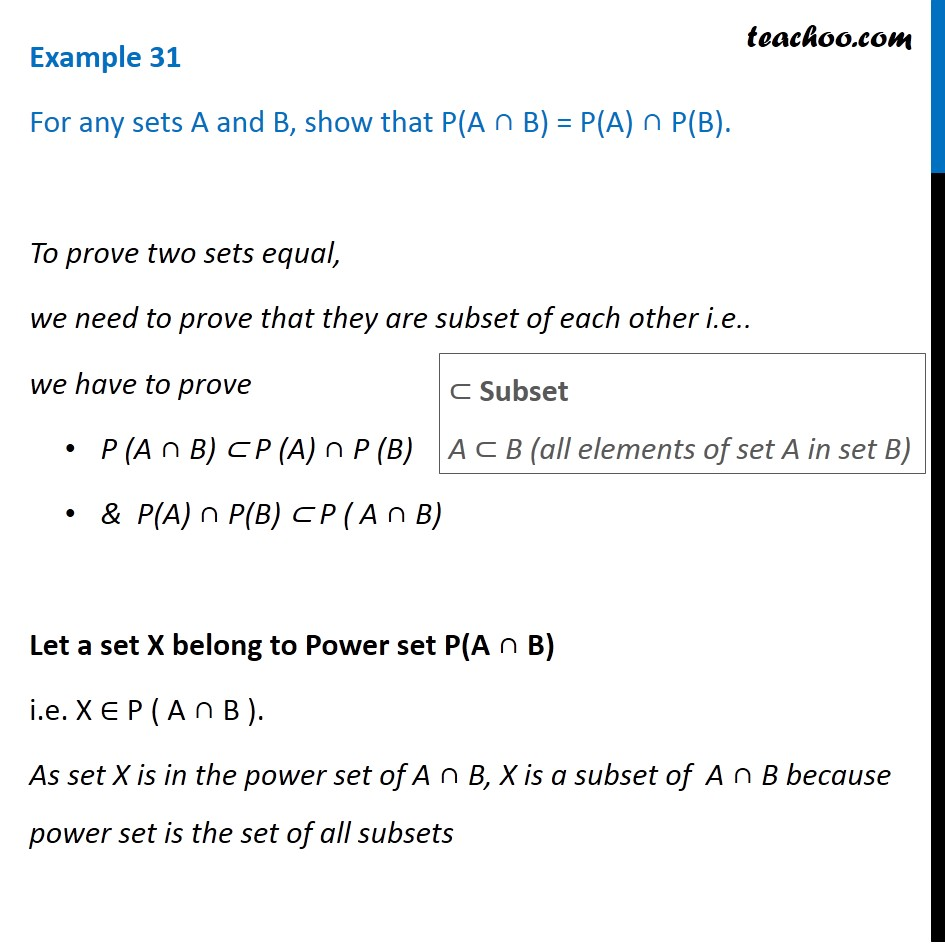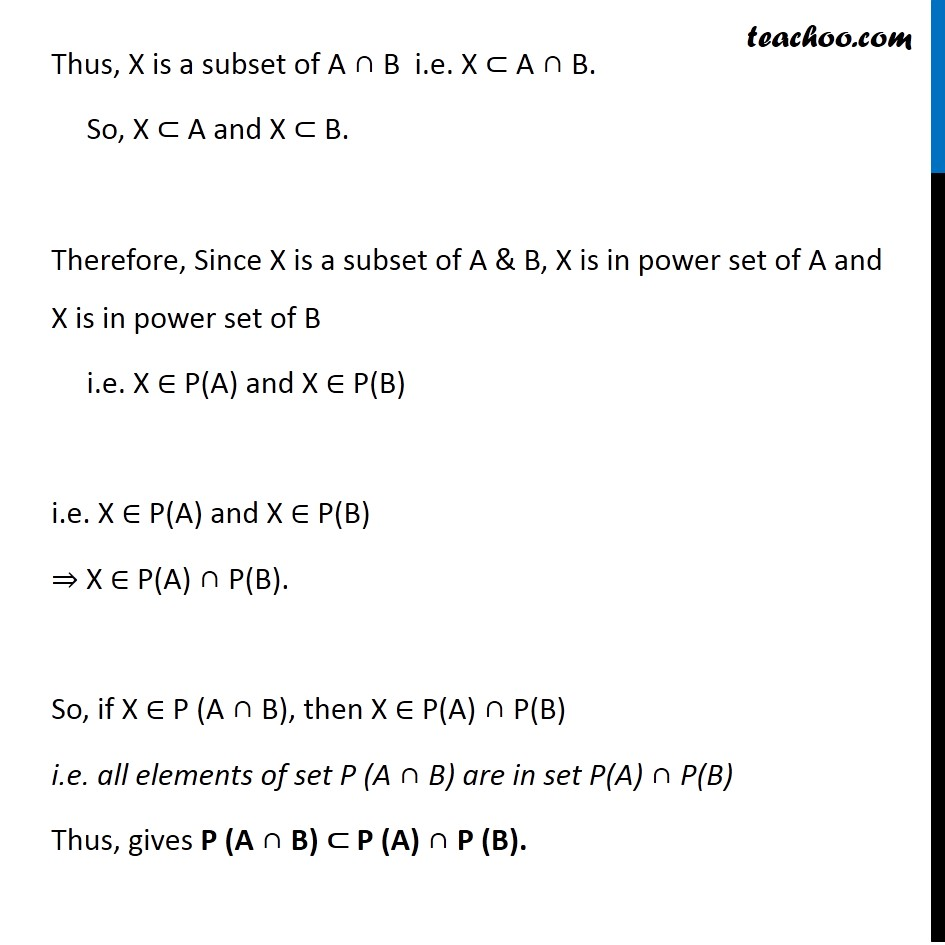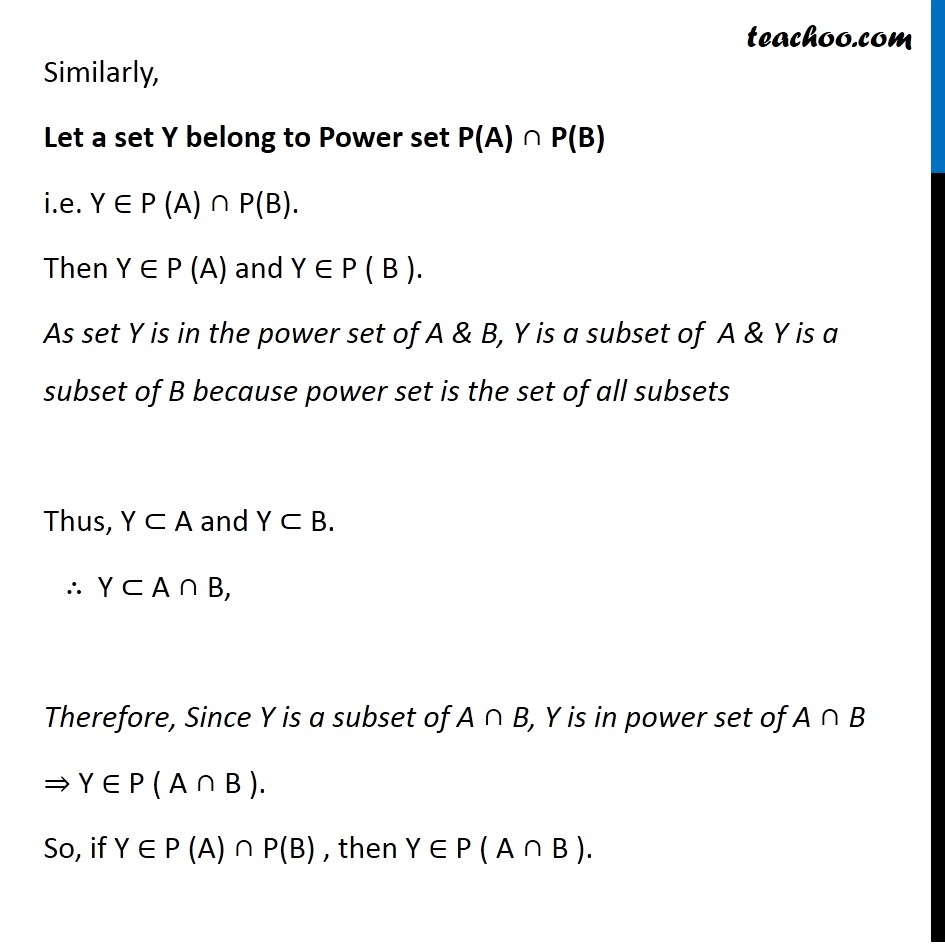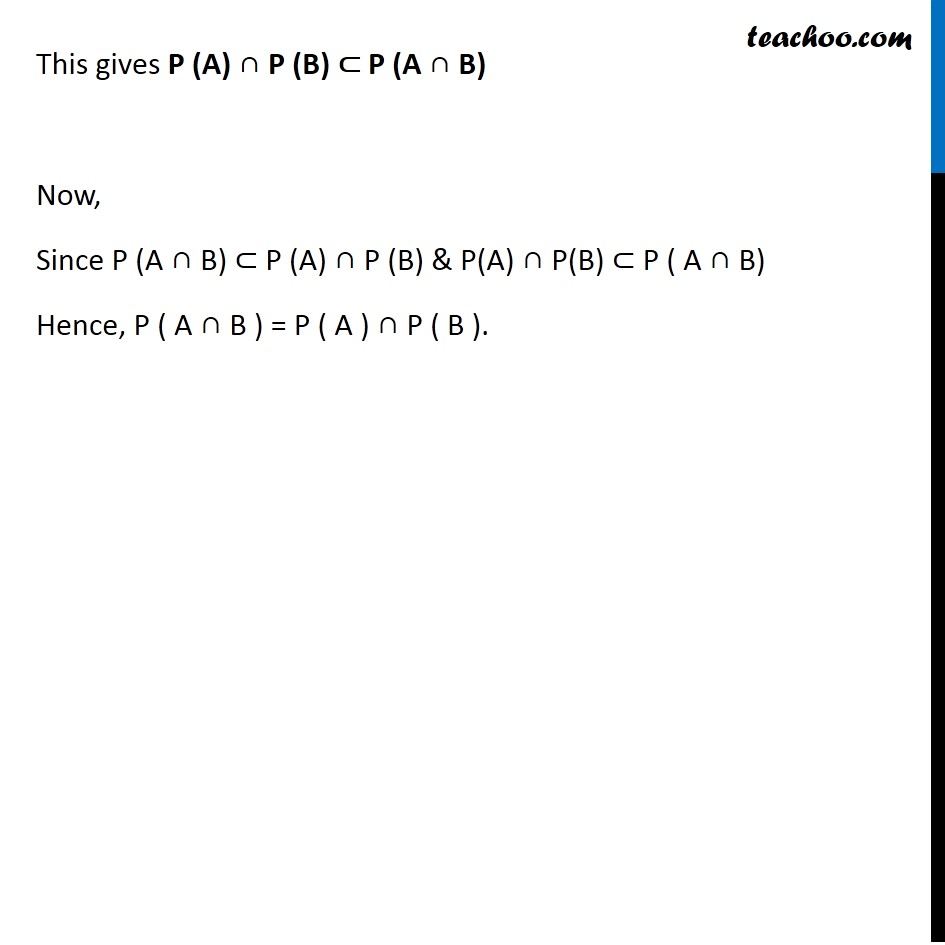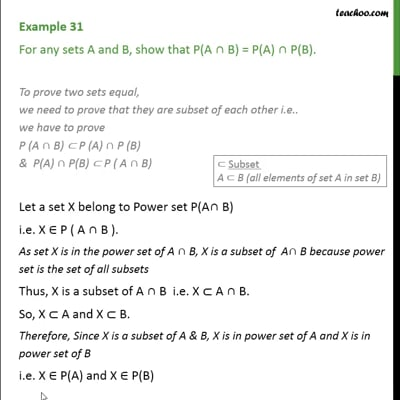This video is only available for Teachoo black users

Learn in your speed, with individual attention - Teachoo Maths 1-on-1 Class

### Transcript

Question 6 For any sets A and B, show that P(A ∩ B) = P(A) ∩ P(B). To prove two sets equal, we need to prove that they are subset of each other i.e.. we have to prove P (A ∩ B) ⊂ P (A) ∩ P (B) & P(A) ∩ P(B) ⊂ P ( A ∩ B) Let a set X belong to Power set P(A ∩ B) i.e. X ∈ P ( A ∩ B ). As set X is in the power set of A ∩ B, X is a subset of A ∩ B because power set is the set of all subsets ⊂ Subset A ⊂ B (all elements of set A in set B) Thus, X is a subset of A ∩ B i.e. X ⊂ A ∩ B. So, X ⊂ A and X ⊂ B. Therefore, Since X is a subset of A & B, X is in power set of A and X is in power set of B i.e. X ∈ P(A) and X ∈ P(B) i.e. X ∈ P(A) and X ∈ P(B) ⇒ X ∈ P(A) ∩ P(B). So, if X ∈ P (A ∩ B), then X ∈ P(A) ∩ P(B) i.e. all elements of set P (A ∩ B) are in set P(A) ∩ P(B) Thus, gives P (A ∩ B) ⊂ P (A) ∩ P (B). Similarly, Let a set Y belong to Power set P(A) ∩ P(B) i.e. Y ∈ P (A) ∩ P(B). Then Y ∈ P (A) and Y ∈ P ( B ). As set Y is in the power set of A & B, Y is a subset of A & Y is a subset of B because power set is the set of all subsets Thus, Y ⊂ A and Y ⊂ B. ∴ Y ⊂ A ∩ B, Therefore, Since Y is a subset of A ∩ B, Y is in power set of A ∩ B ⇒ Y ∈ P ( A ∩ B ). So, if Y ∈ P (A) ∩ P(B) , then Y ∈ P ( A ∩ B ). This gives P (A) ∩ P (B) ⊂ P (A ∩ B) Now, Since P (A ∩ B) ⊂ P (A) ∩ P (B) & P(A) ∩ P(B) ⊂ P ( A ∩ B) Hence, P ( A ∩ B ) = P ( A ) ∩ P ( B ).